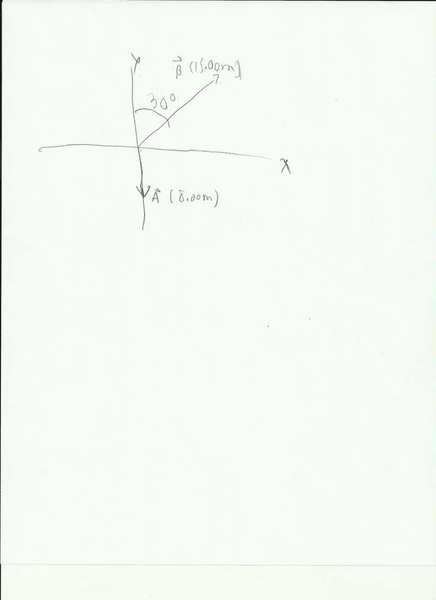# Find the Sum of Two Vectors

• realism877

## Homework Statement

Vector a =8
vector b=15

the solution is 9 and theta is 34 degrees

I want to know how did they come up with that solution.

## The Attempt at a Solution

You are either missing information, or neglecting to post it.

A vector has a magnitude and a direction. What you posted for a and b has the magnitude part down, but is missing direction.

Is this all you were given?

(this will likely be moved -- but I'm not an admin so oh well for now)

You are either missing information, or neglecting to post it.

A vector has a magnitude and a direction. What you posted for a and b has the magnitude part down, but is missing direction.

Is this all you were given?

(this will likely be moved -- but I'm not an admin so oh well for now)

Here is the image of the solutionLast edited:
Vector a =8
vector b=15

Are you sure you wrote this correctly? Is this magnitude? The actual vector? Euclidean vectors have a magnitude and a direction. I guess you could have vectors in R1, where there's only two directions (positive and negative).

However, the presence of an angle and an incorrect magnitude suggest to me that this is not the case. You need to elaborate.

We have to find the magnitude and direction of the sum of vector A and B.

Ok, so how do you typically add vectors together?

Yes

And get the angle

Do I use the law of sines or just the pythogrean theorem?

I know how to get 9 as the solution. HOwever, I don't know how they got 34 degrees as a solution.

You need to express each vector in terms of x and y (or horizontal and vertical) components. You should have either been shown this in your class, or it would be in your textbook as well.

I got how to add them, but how do I find the difference?

I need help finding the angles. How do I do that?

I know I have to put b in the negative direction. Which I did, but what is its angle? What is the angle of a?

Last edited:
I got how to add them, but how do I find the difference?

I need help finding the angles. How do I do that?
tanθ = (y component of vector) divided by (x component of vector)

I know I have to put b in the negative direction. Which I did, but what is its angle? What is the angle of a?
The angle of b will change by 180 degrees when you put it in the opposite direction.
From the figure you posted earlier, a points straight down. That would be either 270 degrees or -90 degrees with respect to the +x direction.